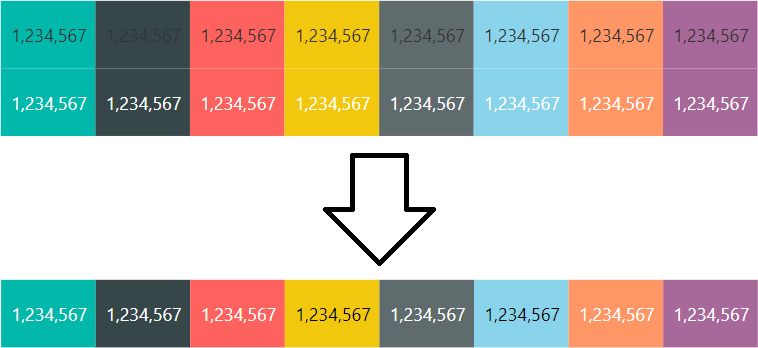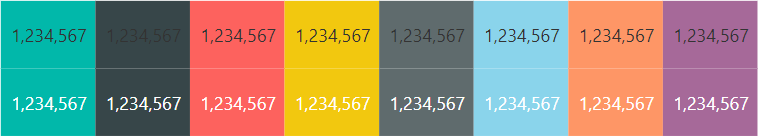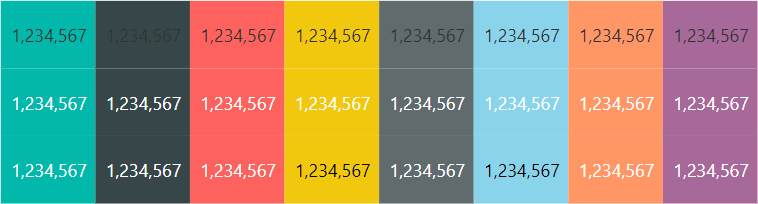# Choosing font color based on background color in Power BIIn August 2018, the Power BI team has released a new feature: conditional formatting by field value. This feature works for both background colors and font colors. Because different background colors require different font colors for text to be legible,  it makes sense to use both at the same time. In this blog post, I am showing how you can dynamically choose the font color depending on the background color.

First of all, I’d like to illustrate the problem by using the default Power BI color palette with black and white font colors:Notice how with the font color fixed, the numbers are sometimes a bit difficult to read. Black font reads okay when the background is light blue, but when it’s dark grey, it’s hardly legible. White works well for darker colors, but not for the lighter ones. Therefore, we need a dynamic font color selection.

Here is one way to solve this problem with DAX:

``````Font Color =
VAR HexCode = UPPER ( {Hex color code} )
VAR SafeHex =
IF (
AND ( LEN ( HexCode ) = 7, LEFT ( HexCode, 1 ) = "#" ),
HexCode,
"#FFFFFF"
)
VAR Digits =
SELECTCOLUMNS (
GENERATESERIES ( 1, 6 ),
"Color Number",
VAR Digit = MID ( SafeHex, [Value] + 1, 1 )
RETURN
IFERROR ( VALUE ( Digit ), UNICODE ( Digit ) - 55 ),
"Base Multiplier", IF ( ISODD ( [Value] ), 16, 1 ),
"Color Multiplier", SWITCH (
TRUE (),
[Value] <= 2, 0.299, -- Red
[Value] <= 4, 0.587, -- Green
[Value] <= 6, 0.114  -- Blue
)
)
VAR Intensity =
SUMX (
Digits,
[Color Number] * [Base Multiplier] * [Color Multiplier]
)
RETURN
IF ( Intensity <= 186, "white", "black" )``````

To make the code above work, simply replace the yellow bit with your background color measure.

Here’s what the formula does:

1. VAR HexCode makes sure all letters, if any, are uppercase — we will need that later.
2. VAR SafeHex ensures the color code is of the expected format: this formula only works with full hex codes, such as #ff3333. Shorthand hex codes, such as #f33, and color names, will not work with this formula.
3. VAR Digits explodes a hex code into a table with six rows, one for each digit, with hexadecimal digits converted and stored in the Color Number column.
• The UNICODE function helps us with converting letters into numbers. For example, the Unicode number of letter A is 65, and it should be 10 when we convert from hex to dec, so we subtract 55.
• Note that the Unicode number of letter a is 97 — this is exactly why we use uppercase letters only.
4. Adds a multiplier for hexadecimal to decimal conversion (the Base Multiplier column): we need to multiply every odd digit by 16 for proper conversion.
5. Adds a multiplier for color intensities (the Color Multiplier column): I just got these values from Mark Ransom on Stack Overflow.
6. In VAR Intensity, we multiply all values and sum them to calculate the total color intensity.
7. Finally, if the total intensity is greater than 186, the font color should be black, otherwise white.

If we now use the measure for font color conditional formatting, the result is as follows (third row):The values are now easy to read regardless of the background color.

• I was just thinking about that contrast issue when I saw the August release. You came up with a very smart solution!

• David Eldersveld

Did you read Mark’s edit on the same Stack Overflow post about using luminance to compare contrast instead of using intensity as in his original formula? A few of these combinations as calculated don’t pass WCAG Level AA for contrast ratio of 4.5, whereas a calculation based on luminance would provide the best option.

• Yes, this was the exact reason why I wrote “here is one way” — I do plan on re-writing this to make it W3C-compliant. Thanks for explaining the implications! This formula is part of something much greater I am working on these days 🙂

• Hadi, have you tried replacing `{Hex color code}` with the measure (not a hard-coded value) that returns your background color? If yes, can you please clarify what you mean by “we are restrict to provide only one hexa-color code”?